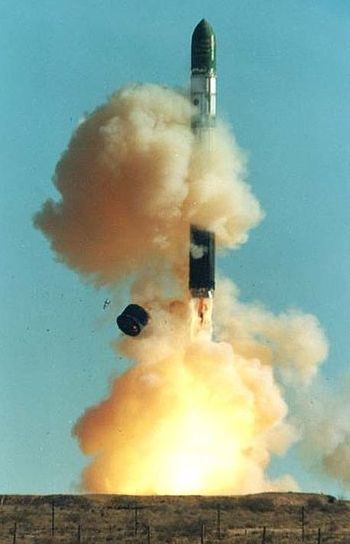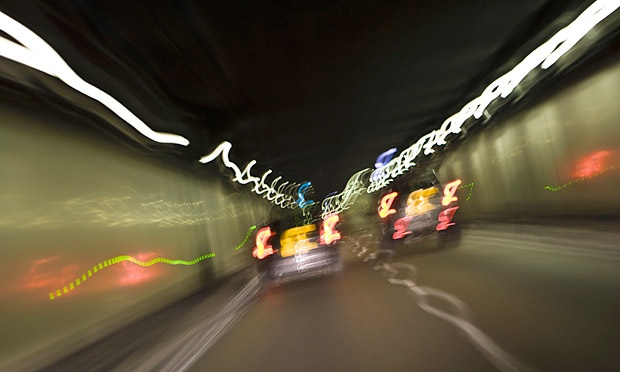Classical Mechanics

# 1D Dynamics: Level 1-2 ChallengesImage credit: ISC Kosmotras

Orbital rockets use liquid propellants, often liquid hydrogen + oxygen, to get their large mass off the ground. Burning liquid oxygen produces a tremendous amount of thrust. If the total mass of a rocket, payload, and fuel is $2\times 10^6$ kg, what is the necessary thrust (in Newtons) to start the rocket moving from its launch platform?

Details: the acceleration of gravity is $-9.8\text{ m/s}^2$ on the platform.

A very icy man who likes to travel decides that he wants to travel around the country in style. He buys a train to do his traveling on and gives it all sorts of neat cars - one car has a pool, one has a movie theatre, etc. His pride and joy is the ice rink car, a 30 meter long train car with the floor covered in ice so he can ice skate. Our icy guy is standing in the middle of his ice rink car, thinking what an icy guy he is, when his train begins to accelerate at $2~m/s^2$. How long does it take (in seconds) before our icy guy slides smack into the back of the train car?

Moral of the story: unlike laws made by people, the laws of physics apply equally to everyone, even people who love broom ball.

Details and assumptions

• The static and kinetic coefficients of friction between the ice guy's shoes and the ice is 0.1.
• The acceleration of gravity is $-9.8~m/s^2$.

Unfortunately, cats fall out of windows in cities sometimes. In a famous article, The New York Times notes that the likelihood that a cat survives a fall goes down as the fall distance increases (expected) but then goes back up at very large distances (perhaps unexpected). If the statistics are correct, then there should be some physical reason this occurs. Some have suggested that terminal velocity and cat biology come into play. The article above indicates that cats have a terminal velocity of 60 miles per hour (mph). If we model the drag force $F_d$ on a cat as

$F_d=\frac {1} {2} k A v^2$

where $A$ is the cross-sectional area of the cat, $v$ is its velocity and $k = 1 \text{ kg/m}^3$, what is the cross-sectional area in $\mbox{m}^2$ of a $5 \text{ kg}$ cat with a terminal velocity of $60 \text{ mph}$?

Details and assumptions

• The acceleration of gravity is $-9.8~\mbox{m/s}^2$

• $1 \text{ mile} = 1.6 \text{ km}$The snow pile in the picture above is in?In order to stop a car in shortest distance on a horizontal road, one should

(A) apply the brakes very hard so that the wheels stop rotating

(B) apply the brakes hard enough to just prevent slipping

(C) pump the brakes (press and release)

(D) shut the engine off and not apply brakes

AYWC?

×

Problem Loading...

Note Loading...

Set Loading...